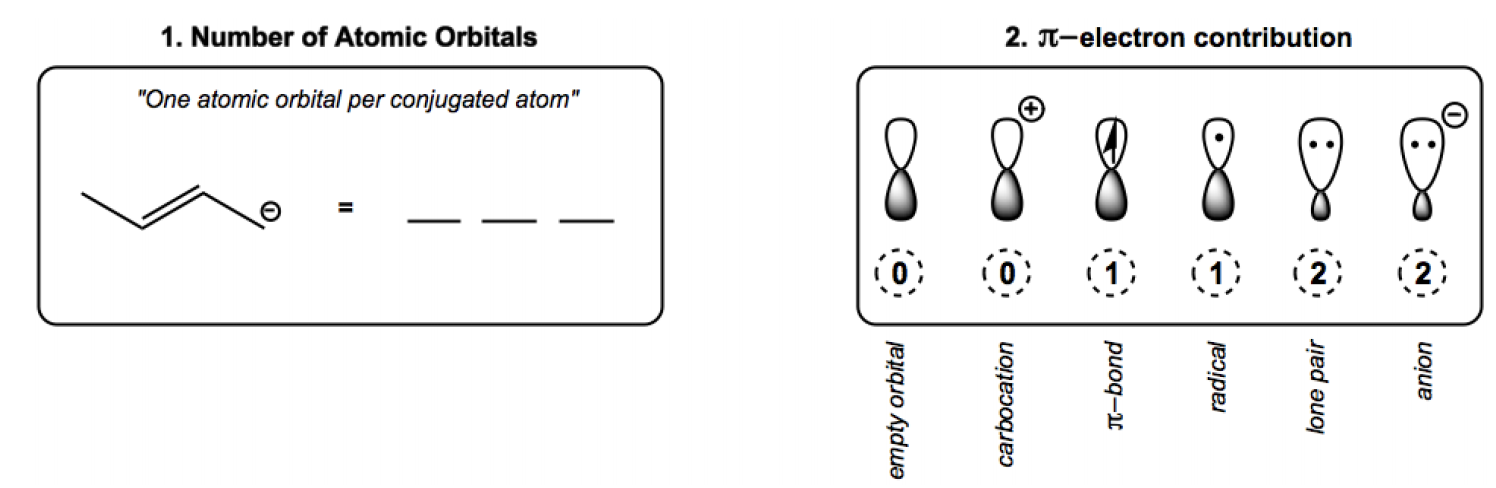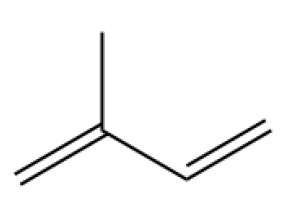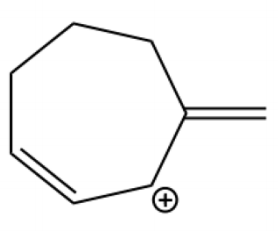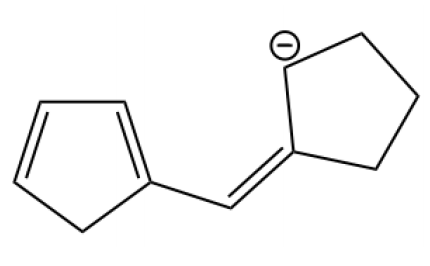## Organic Chemistry

Learn the toughest concepts covered in Organic Chemistry with step-by-step video tutorials and practice problems by world-class tutors.

16. Conjugated Systems

# Drawing Atomic Orbitals

Get out your pencil (and eraser) because we are about to learn how to draw atomic orbitals.

Two Rules:

1.  The # of atomic orbitals = the # of conjugated atoms
2. You need to know what type of pi electron contribution each type of non-bonding orbital will have
1
concept

## Two Rules of Drawing Atomic Orbitals2m
Play a video:2
example

## Supply Atomic Orbitals1m
Play a video:
Let's do a first. So a, it says, provide the correct atomic orbital for the following conjugated molecules. So how? Let's start with number one. How many atomic orbital should there be on this molecule? Well, even though I see five atoms total total, the conjugated part is just this right, Because those are the only atoms that can resonate. So that means that I should have 1234 atomic orbital's. And I'm gonna draw them like this. Cool. Now I have to figure out how Maney pie electrons are being donated throughout the entire molecule. Okay, Now, one way to do this is to just count it up the way that I said, which is that every pi bon Adam donates one electron. Okay, so right now I have two pi bonds. But that therefore, Adams that inside those two pi bonds, right? So that means that each Adam would donate one electron, meaning that I would have 123 four. Okay, now, another shortcut that you can take that might be helpful is you can also just think that a pie bond total always don't need two electrons over two atoms. So another way you could think of it is that there's two electrons here. 234 electrons three and four are there, So there should be four total. Does that make sense? Because you have to hear. And then you have another two here, giving you a total of four. Okay, so I got to this answer using my definition. But you can also just use the shortcut that ah, pie bond always has two electrons that are being donated. Quote. So let's go ahead and try to try to do the second one on your own, and then I'll show you how.3
example

## Supply Atomic Orbitals1m
Play a video:
So for the second one, provide the correct atomic orbital's. What I see is that the conjugated part of the molecule is this right. So how many atoms is that? It appears to be 12345 I have five. So let's draw that out. 12345 Cool. So that means that now I have tow count up my pie Electrons. Now my pie electrons We know that double bonds donate to each, so I'm gonna have to here. I'm gonna have to Here. I know that cat ions donate zero, right? So that means the total sum should be equal Toe four. I have four pi electrons total. Now I just have to put them in the right spots. And you would actually try toe line it up based on the order of these of these atoms. What I would do is I would do this one to nothing. 12 And what that shows me is that there are four pie. There are four pi conjugated electrons inside of five atomic orbital's. These. This would be the correct atomic orbital diagram for this molecule. Cool. Let's keep going4
example

## Supply Atomic Orbitals1m
Play a video: